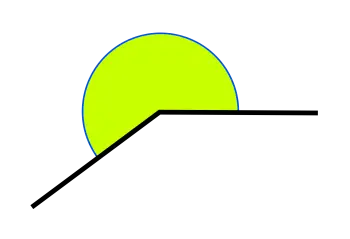# Concave Angle - Measure and Definition of Concave PolygonsIn this article, you will discover everything you need to know about concave angles, their definition, and the properties of this element of geometry

This definition of concave angle corresponds to the classification of angles according to their angle. According to this classification, the types of angles can be:

• Flat angles (180 degrees)

• Convex angle (less than 180 degrees)

• Concave angle (more than 180 degrees)

• Full angle (360 degrees)

## What Is a Concave Angle?

A concave angle is a type of angle that is defined by its opening. The essential characteristic to form a concave angle is that it measures more than 180°.

These types of angles can also be called receptive angles or reflex angles.

## What Is a Concave Polygon?

A concave polygon is a polygon with at least one interior angle greater than 180 degrees. In other words, concave polygons are irregular polygons with at least one "reentrant" vertex, meaning that the vertex points inwards towards the center of the polygon. This is an essential property of a concave polygon.

The difference between a convex and concave polygon is that in convex polygons, all vertex points outwards, and in a concave one, at least one internal angle points inwards.

A triangle can not be concave in any case because the sum of interior angles adds up 180.

There are many different applications for concave polygons. One everyday use is in architecture, where concave shapes can add interest and variety to a building's facade. Another benefit is graphic design, where concave polygons can create attractive negative spaces within a composition.

## What Is the Measure of a Concave Angle?

A concave angle measures more than 180º or π rad (PI radians) and less than 360º or 2π rad.

It is measured in degrees by the length of its arc. A concave angle can never be acute, it is always obtuse because it always measures more than 90 degrees.

Acute angles are those that measure less than 90 degrees.

## Characteristics of a Concave Angle

The main features that define this type of angle are:

• They have more than 180 degrees or PI radians, so it has an open shape visually.

• A concave angle cannot have a complementary. The sum of the complementary angles must add up to 90 so that this condition cannot be fulfilled due to the definition of this type of angle.

• In a regular polygon, all the exterior angles of the geometric figure are concave and the interior angles are convex.

• These angles cannot have an adjacent angle. Two adjacent angles must add up to 180º, a condition that cannot be met because the first angle already measures more than 180.

## What Is the Difference Between a Concave Angle and a Convex Angle?

The difference between a convex and a concave angle is determined by the angle of the ope. In the case of the convex angle, the angle is always less than 180 degrees, while the concave angles measure more than 180 degrees.

When the ends of two segments meet at a point, two angles are formed, one concave and one convex on the other side.

Author:

Published: March 12, 2023
Last review: March 12, 2023